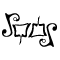# Math Is Fun Forum

Discussion about math, puzzles, games and fun.   Useful symbols: ÷ × ½ √ ∞ ≠ ≤ ≥ ≈ ⇒ ± ∈ Δ θ ∴ ∑ ∫  π  -¹ ² ³ °

You are not logged in.

## #1 2005-10-22 17:44:25

ryos
MemberRegistered: 2005-08-04
Posts: 394

### Find cubic function from extrema

Find a cubic function of the form f(x) = ax^3 + bx^2 + cx  which has a local maximum at f(1) = 0 and a local minimum at f(3) = -2.

I'm stumped out of my mind. I'm pretty sure that both b and c must be negative, but beyond that, no matter how I munge and funge that function, I can't get it to behave. Is there some voodoo calculus trick that I'm missing here?

(I've tried solving the coefficients as a system of equations, but that didn't work. Every time I got it down to one variable with substitutions, the variable would cancel out, leaving me with something stupid like -2=-2. But, maybe I did it wrong.)

Thanks guys.

El que pega primero pega dos veces.

Offline

## #2 2005-10-22 18:50:03

MathsIsFunRegistered: 2005-01-21
Posts: 7,710

### Re: Find cubic function from extrema

f(x) = ax^3 + bx^2 + cx
f'(x) = 3ax^2 + 2bx + c

Maxima and Minima are where slope=0, ie f'(x)=0

Where slope=0:
x=1: 3a + 2b + c = 0
x=3: 27a + 6b + c = 0

Now, we also know that f(1) = 0 and f(3) = -2:

a + b + c = 0
27a + 9b + 3c = -2

So, now it is substitution time"The physicists defer only to mathematicians, and the mathematicians defer only to God ..."  - Leon M. Lederman

Offline

## #3 2005-10-22 19:14:41

MathsIsFunRegistered: 2005-01-21
Posts: 7,710

### Re: Find cubic function from extrema

3 unknowns and 4 equations ...

27a + 6b + c = 0
27a + 9b + 3c = -2

reduces to 3b+2c=-2, and then b=-2/3 - (2/3)c

a + b + c = 0
==> a = -b-c
==> a = -(-2/3 - (2/3)c)-c
==> a = 2/3 + (2/3)c - c
==> a = 2/3 - (1/3)c

3a + 2b + c = 0
==> 3(2/3 - (1/3)c) + 2(-2/3 - (2/3)c) + c = 0
==> (2 - c) + (-4/3 - (4/3)c) + c = 0
==> 2 - c  -4/3 - (4/3)c + c = 0
==> 2 -4/3 - (4/3)c = 0
==> 2/3 - (4/3)c = 0
==> 2 - 4c = 0
==> 2 = 4c
==> c = 1/2

Now, I haven't made a mistake (and my mistake rate is about 10%, so it is likely)

b = -2/3 - (2/3)c = -2/3 - (1/3) = -1
a = 2/3 - (1/3)c = 2/3 - (1/6) = 1/2

Hmm, must have made a miscalc ... this seems odd, not working out ...

Maybe we are missing the "d" term: f(x) = ax^3 + bx^2 + cx + d

I will work on it later today, ryos.

"The physicists defer only to mathematicians, and the mathematicians defer only to God ..."  - Leon M. Lederman

Offline

## #4 2005-10-22 20:40:27

MathsIsFunRegistered: 2005-01-21
Posts: 7,710

### Re: Find cubic function from extrema

OK, I did it all on paper, and you do need the "d" term.

The results are:

a=1/2
b=-3
c=4.5
d=-2

I have to go now, but can do a better posting later.

"The physicists defer only to mathematicians, and the mathematicians defer only to God ..."  - Leon M. Lederman

Offline

## #5 2005-10-22 21:39:12

MathsIsFunRegistered: 2005-01-21
Posts: 7,710

### Re: Find cubic function from extrema

OK, this is what I had on paper:

27a + 9b + 3c + d = -2
a + b + c + d = 0

Subtract and get: 26a + 8b + 2c = -2

Now you have 3 equations and 3 unknowns:

(1) 3a + 2b + c = 0
(2) 27a + 6b + c = 0
(3) 26a + 8b + 2c = -2

Using (1): 3a + 2b + c = 0 ==> c = -3a -2b
Combine with (2): 27a + 6b + (-3a -2b) = 0
==> 24a + 4b = 0
==> a = -(1/6)b

Substitute into (3) gets:
26a + 8b + 2c = -2
==> (-26/6)b + 8b + 2c = -2
==> (22/6)b + 2c = -2
==> c = -1 - (22/12) b

Putting all that in (1) gets:
3a + 2b + c = 0
==> 3(-1/6) b + 2b + (-1 - (22/12) b) = 0
==> (-3/6 + 2 - 22/12)b = 1
==> b = -3

Knowing b, we can calculate
a = -(1/6) b = 1/2
c = -1 - (22/12) b = 4.5

and then calculate d:
a + b + c + d = 0
0.5 + (-3) + 4.5 + -2 = 0

DONE

"The physicists defer only to mathematicians, and the mathematicians defer only to God ..."  - Leon M. Lederman

Offline

## #6 2005-10-23 15:07:59

ryos
MemberRegistered: 2005-08-04
Posts: 394

### Re: Find cubic function from extrema

Thanks, you're a lifesaver. I had forgotten that you need at least as many equations as unknowns, and didn't think to use the derivatives.

El que pega primero pega dos veces.

Offline